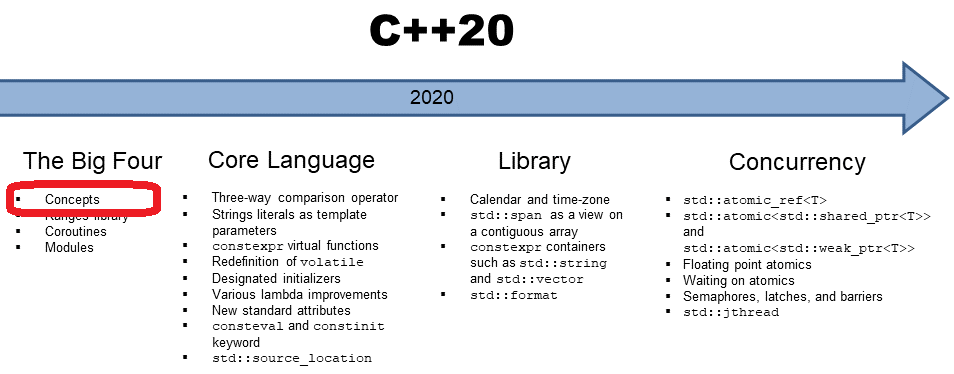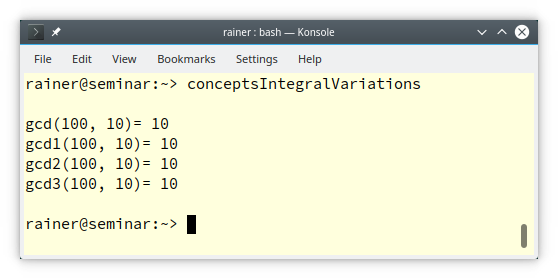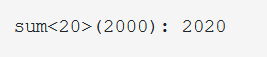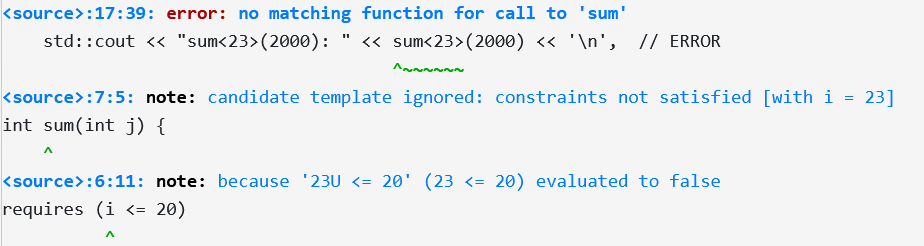# Using Requires Expression in C++20 as a Standalone Feature

In my last post “Defining Concepts with Requires Expressions“, I exemplified how you can use requires expressions to define concepts. Requires expressions can also be used as a standalone feature when a compile-time predicate is required.Typical use cases for compile-time predicates are` static_assert`, `constexpr if`, or a requires clause. A compile–time predicate is an expression that returns at compile time a boolean. Let me start this post with C++11.

## `static_assert`

`static_assert` requires a compile-time predicate, and a message is displayed when the compile-time predicate fails. With C++17, the message is optional. With C++20, this compile-time predicates can be a requires expression.

```// staticAssertRequires.cpp

#include <concepts>
#include <iostream>

struct Fir {                   // (4)
int count() const {
return 2020;
}
};

struct Sec {
int size() const {
return 2021;
}
};

int main() {

std::cout << '\n';

Fir fir;
static_assert(requires(Fir fir){ { fir.count() } -> std::convertible_to<int>; });     // (1)

Sec sec;
static_assert(requires(Sec sec){ { sec.count() } -> std::convertible_to<int>; });     // (2)

int third;
static_assert(requires(int third){ { third.count() } -> std::convertible_to<int>; }); // (3)

std::cout << '\n';

}
```

The requires expressions (lines 1, 2, and 3) check if the object has a member function `count` and if its result is convertible to` int`. This check is only valid for the class `First` (lines 4). On the contrary, the checks in lines (2) and (3) fail.Maybe, you want to compile code depending on a compile-time check. In this case, the C++17 feature `constexpr if` combined with requires expressions provides you with the necessary tool.

## `constexpr if`

`constexpr if` allows it to compile source code conditionally. For the condition, the requires expression comes into play. All branches of the if statement have to be valid.

Thanks to `constexpr if`, you can define functions that inspect their arguments at compile time and generate different functionality based on their analysis.

```// constexprIfRequires.cpp

#include <concepts>
#include <iostream>

struct First {
int count() const {
return 2020;
}
};

struct Second {
int size() const {
return 2021;
}
};

template <typename T>
int getNumberOfElements(T t) {

if constexpr (requires(T t){ { t.count() } -> std::convertible_to<int>; }) {   // (1)
return t.count();
}
if constexpr (requires(T t){ { t.size() } -> std::convertible_to<int>; }) {    // (2)
return t.size();
}
else return 42;                                                                // (3)

}

int main() {

std::cout << '\n';

First first;
std::cout << "getNumberOfElements(first): "  << getNumberOfElements(first) << '\n';

Second second;
std::cout << "getNumberOfElements(second): "  << getNumberOfElements(second) << '\n';

int third;
std::cout << "getNumberOfElements(third): " << getNumberOfElements(third) << '\n';

std::cout << '\n';

}
```

##Modernes C++ Mentoring

Be part of my mentoring programs:

• "Fundamentals for C++ Professionals" (open)
• "Design Patterns and Architectural Patterns with C++" (open)
• "C++20: Get the Details" (reopens December 2023)
• Do you want to stay informed about my mentoring programs: Subscribe via E-Mail.

Lines (1) and (2) are crucial in this code example. In line (1), the requires expressions determine if the variable `t` has a member function `count` that returns an `int`. Accordingly, line (2) determines if the variable` t` has a member function `size`. The else statement in line (3) is applied as a fallback.## Requires Clause

First of all, I have to answer the question: What is a requires clause?

There are essentially four ways to use a concept, such as `std::integral`.

```// conceptsIntegralVariations.cpp

#include <concepts>
#include <type_traits>
#include <iostream>

template<typename T>                          // (1)
requires std::integral<T>
auto gcd(T a, T b) {
if( b == 0 ) return a;
else return gcd(b, a % b);
}

template<typename T>                          // (2)
auto gcd1(T a, T b) requires std::integral<T> {
if( b == 0 ) return a;
else return gcd1(b, a % b);
}

template<std::integral T>                     // (3)
auto gcd2(T a, T b) {
if( b == 0 ) return a;
else return gcd2(b, a % b);
}

auto gcd3(std::integral auto a, // (4)
std::integral auto b) {
if( b == 0 ) return a;
else return gcd3(b, a % b);
}

int main(){

std::cout << '\n';

std::cout << "gcd(100, 10)= "  <<  gcd(100, 10)  << '\n';
std::cout << "gcd1(100, 10)= " <<  gcd1(100, 10)  << '\n';
std::cout << "gcd2(100, 10)= " <<  gcd2(100, 10)  << '\n';
std::cout << "gcd3(100, 10)= " <<  gcd3(100, 10)  << '\n';

std::cout << '\n';

}
```

Thanks to the header` <concepts`> I can use the concept `std::integral`. The concept is fulfilled if `T` it is integral. The function name `gcd` stands for the greatest-common-divisor algorithm based on the Euclidean algorithm.

Here are the four ways to use concepts:
1.  Requires clause (line 1)
2. Trailing requires clause (line 2)
3. Constrained template parameter (line 3)
4. Abbreviated function template (line 4)
For simplicity reasons, each function template returns `auto`. There is a semantic difference between the function templates` gcd, gcd1, gcd2`, and the function `gcd3`. In the case of `gcd, gcd1, or gcd2`, arguments `a` and `b` must have the same type. This does not hold for the function `gcd3`. Parameters` a` and` b` can have different types but must both fulfill the concept `std::integral.`The functions `gcd` and `gcd1` use requires clauses.

There is an interesting fact about requires clauses. You can use any compile-time predicate as an expression. I check in the following requirces clause if an int as a non-type template parameter is smaller than 20.

```// requiresClause.cpp

#include <iostream>

template <unsigned int i>
requires (i <= 20)             // (1)
int sum(int j) {
return i + j;
}

int main() {

std::cout << '\n';

std::cout << "sum<20>(2000): " << sum<20>(2000) << '\n',
// std::cout << "sum<23>(2000): " << sum<23>(2000) << '\n',  // ERROR

std::cout << '\n';

}
```

The compile-time predicate used in line (1) exemplifies an interesting point: the requirement is applied to the non-type` i`, and not on a type as usual.When you use the commented-out line in the `main` program, the clang compiler reports the following error:Here are more details about non-type template parameters: “Alias Templates and Template Parameters“.

Typically, you use a concept in a requires clause, but there is more: `requires requires` or anonymous concepts

### `requires requires` or anonymous concepts

You can define an anonymous concept and directly use it. In general, you should not do it. Anonymous concepts make your code hard to read, and you cannot reuse your concepts.

```template<typename T>
requires requires (T x) { x + x; }
auto add(T a, T b) {     return a + b; }
```

The function template defines its concept as ad-hoc. The function template `add `uses a requires expression (`requires(T x) { x + x; }` ) inside a requires clause. The anonymous concept is equivalent to the following concept Addable.

```template<typename T>
concept Addable = requires (T a, T b) {
a + b;
};
```

Consequentially, the following four implementations of the function template `add` are equivalent to the previous one:

```template<typename T>  // requires clause
auto add(T a, T b) {
return a + b;
}

template<typename T>  // trailing requires clause
return a + b;
}

template<Addable T>   // constrained template parameter
return a + b;
}
// abbreviated function template
return a + b;
}
```

As a short reminder: The last implementation based on abbreviated function templates syntax can deal with values having different types.

Again, I want to emphasize it: Concepts should encapsulate general ideas and give them a self-explanatory name for reuse. They are invaluable for maintaining code. Anonymous concepts read more like syntactic constraints on template parameters and should be avoided.

## What’s next?

Using a concept in` static_assert(Concept<T>`) tests whether the type` T` fulfills the concept. Let’s see how we can use this in my next post.

Thanks a lot to my Patreon Supporters: Matt Braun, Roman Postanciuc, Tobias Zindl, G Prvulovic, Reinhold Dröge, Abernitzke, Frank Grimm, Sakib, Broeserl, António Pina, Sergey Agafyin, Андрей Бурмистров, Jake, GS, Lawton Shoemake, Jozo Leko, John Breland, Venkat Nandam, Jose Francisco, Douglas Tinkham, Kuchlong Kuchlong, Robert Blanch, Truels Wissneth, Kris Kafka, Mario Luoni, Friedrich Huber, lennonli, Pramod Tikare Muralidhara, Peter Ware, Daniel Hufschläger, Alessandro Pezzato, Bob Perry, Satish Vangipuram, Andi Ireland, Richard Ohnemus, Michael Dunsky, Leo Goodstadt, John Wiederhirn, Yacob Cohen-Arazi, Florian Tischler, Robin Furness, Michael Young, Holger Detering, Bernd Mühlhaus, Matthieu Bolt, Stephen Kelley, Kyle Dean, Tusar Palauri, Dmitry Farberov, Juan Dent, George Liao, Daniel Ceperley, Jon T Hess, Stephen Totten, Wolfgang Fütterer, Matthias Grün, Phillip Diekmann, Ben Atakora, Ann Shatoff, Rob North, Bhavith C Achar, and Marco Parri Empoli.

Thanks, in particular, to Jon Hess, Lakshman, Christian Wittenhorst, Sherhy Pyton, Dendi Suhubdy, Sudhakar Belagurusamy, Richard Sargeant, Rusty Fleming, John Nebel, Mipko, Alicja Kaminska, Slavko Radman, and David Poole.

 My special thanks to EmbarcaderoMy special thanks to PVS-StudioMy special thanks to Tipi.buildMy special thanks to Take Up Code## Seminars

I’m happy to give online seminars or face-to-face seminars worldwide. Please call me if you have any questions.

### Standard Seminars (English/German)

Here is a compilation of my standard seminars. These seminars are only meant to give you a first orientation.

• C++ – The Core Language
• C++ – The Standard Library
• C++ – Compact
• C++11 and C++14
• Concurrency with Modern C++
• Design Pattern and Architectural Pattern with C++
• Embedded Programming with Modern C++
• Generic Programming (Templates) with C++

#### New

• Clean Code with Modern C++
• C++20

### Contact Me

Modernes C++ Mentoring,Tags:
0 replies